Classrooms Curricula References Textbooks Site Map

## Definitions

Set means the same thing in algebra as it does in English: a collection of objects. When you first start talking about sets in class you usually talk about things like "the set of all dogs" or "the set of students in class today". Sets that are made up of real world objects are easy to understand because they're based on your every day experience. Once you've got the basic idea, the discussion drifts back to mathematics by talking about sets of numbers. These sets can be finite, i.e. they have a specific number of elements, or inifinite. Finite sets are usually defined by listing their elements, for example {1, 3, 5} is the set whose elements are the numbers 1, 3 and 5. Infinite sets usually have to be described using words, for example "the set of all numbers that can be written as a fractoin" or "the set of all numbers between 0 and 1".

There are some special sets of numbers that you should be aware of.

 Name Symbol Description Natural Numbers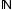The numbers that "occur in nature": 1, 2, 3, 4, ... Whole Numbers The natural numbers and 0: 0, 1, 2, 3, 4, ... Integers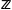The whole numbers and their negatives: ..., -4, -3, -2, -1, 0, 1, 2, 3, 4, ... Rational Numbers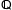Any number that can be written as a fraction. This includes the integers because you can, for example, write 2 as 2 / 1. Algebraic Numbers Any number that can be the solution of a polynomial equation (e.g. x2 - 2 = 0. This set includes all the rational numbers and "roots" such as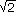. Real Numbers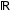Any number including the rational numbers, the algebraic numbers and "irrational numbers" (ones that can't be written as a fraction and that can't be the solution of a polynomial equations, e.g. π).

## Special Symbols

Set theory has its a collection of special symbols for representing ideas like "intersection" and "inclusion". In the examples below assume that A = {1, 2, 3, 4, 5}, B = {-5, -4, -3, -2, -1, 0)} and C = {1, 2, 3, 4, 5, 6, 7, 8}.

 Name Symbol Description Example element of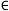The item on the left of the symbol is contained in the set on the right. 1∈A subset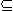All of the elements in the set on the left side of the symbol are also in the set on the right side of the symbol. AC because every number in A is also in C. proper subset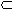All of the elements in the set on the left side of the symbol are also in the set on the right side of the symbol but the set on the right has at least one element that isn't in the set on the left, i.e. the sets aren't equal. You could never say that AA because every number in the set on the left is also in the set on the right. intersection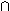the set of all elements that the two sets have in common AC = {1, 2, 3, 4, 5} union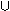the set of all elements that are in either of the two sets, i.e. the two sets combined together into one big set AB = {-5, -4, -3, -2, -1, 0, 1, 2, 3, 4, 5} empty set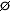the set with no elements AB =because they have no elements in common.

There's a technicality with the subset idea that you should be aware of. You should never say {1} ∈ C. At first glance this might look okay. After all, 1 is an element of the set C, right? Here's the problem: {1} doesn't mean the element 1, it means "the set whose only element is 1". The original set, C, doesn't contain any sets, only numbers, so it's technically incorrect to